# RBI Assistant 2022 Quantitative Aptitude Quiz – 1

## RBI Assistant 2022 Quantitative Aptitude Quiz

Quantitative Aptitude measures a candidate’s numerical ability and problem-solving abilities. It is the most important section of almost all competitive exams. Candidates are often stymied by the complexity of Quantitative Aptitude Questions but if they practice more and more questions, it will become quite simple. So, here we are providing you with the RBI Assistant 2022 Quantitative Aptitude Quiz to enhance your preparation for your upcoming examination. This RBI Assistant 2022 Quantitative Aptitude Quiz is based on the most recent and the latest exam pattern. A detailed explanation for each question will be given in this RBI Assistant 2022 Quantitative Aptitude Quiz. This RBI Assistant 2022 Quantitative Aptitude Quiz is entirely free of charge. This RBI Assistant 2022 Quantitative Aptitude Quiz will assist aspirants in achieving a good score in their upcoming examinations.

Directions (1-5): In each of these questions, two equations (I) and (II) are given. You have to solve both the equations and give answer.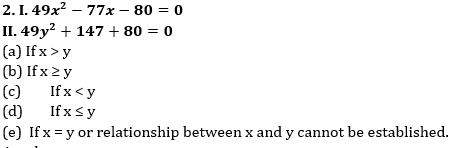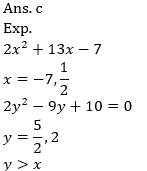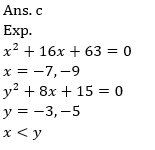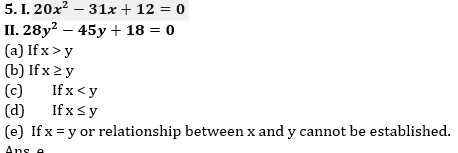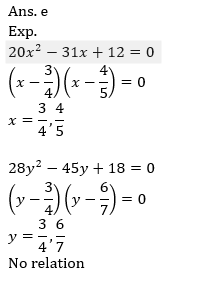Directions (6-10): Read the given data carefully and answer the given question.
A manufacturing unit produces five types of products and Maximum Retail (MRP), Percent discount (% Discount) Selling Price (SP), and Profit Percent (% Profit) on each product given provided some data are missing.6. If cost price of P1 is Rs. 9000. Profit of 70% is gain when it is sold at MRP. Find Profit percent when it is sold at selling Price.
(a) 30%
(b) 25%
(c) 28%
(d) 32%
(e) None of these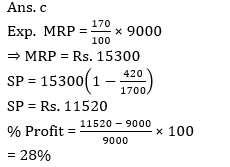7. If MRP of P2 is 71% more than the cost price then find percent discount given P2
(a) 100/11%
(b) 50/3%
(c) 20%
(d) 100/9%
(e) None of these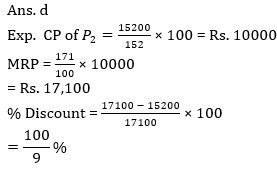8. If cost Price of P2 and P4 are same find overall profit amount on both products together in Rs.
(a) Rs. 7500
(b) Rs. 8200
(c) Rs. 8000
(d) Rs. 8500
(e) None of these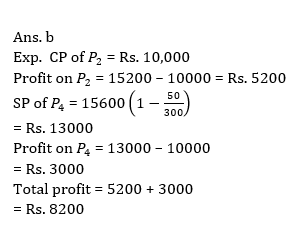9. If MRP is 60% more than the cost price then find the difference between profit and discount on P3.
(a) Rs. 1800
(b) Rs. 3600
(c) Rs. 2700
(d) Rs. 900
(e) None of these10. If percent discount for P5 is 20% more than the discount offered on P3. Find MRP of P5.
(a) Rs. 16000
(b) Rs. 16200
(c) Rs. 15800
(d) Rs. 15900
(e) None of these#### Attempt Quantitative Aptitude Topic Wise Online Test Series

RBI Assistant Prelims Online Test Series 2022

Recommended PDF’s for:

#### Most important PDF’s for Bank, SSC, Railway and Other Government Exam : Download PDF Now

AATMA-NIRBHAR Series- Static GK/Awareness Practice Ebook PDF Get PDF here
The Banking Awareness 500 MCQs E-book| Bilingual (Hindi + English) Get PDF here
AATMA-NIRBHAR Series- Banking Awareness Practice Ebook PDF Get PDF here
Computer Awareness Capsule 2.O Get PDF here
AATMA-NIRBHAR Series Quantitative Aptitude Topic-Wise PDF Get PDF here
AATMA-NIRBHAR Series Reasoning Topic-Wise PDF Get PDF Here
Memory Based Puzzle E-book | 2016-19 Exams Covered Get PDF here
Caselet Data Interpretation 200 Questions Get PDF here
Puzzle & Seating Arrangement E-Book for BANK PO MAINS (Vol-1) Get PDF here
ARITHMETIC DATA INTERPRETATION 2.O E-book Get PDF here
3